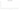# FC

## Comorian Franc

The Comoros' official currency is the franc. Although there have never been any centime denominations produced, it is nominally divisible into 100 centimes.

#### how has the value of the currency changed in the last year?## USD 1 = KMF 466.6

How much is10 US dollars worth inComorian francs?
At the current exchange rate, 10 US dollars is worth 4,666 Comorian francs
How much is50 US dollars worth inComorian francs?
At the current exchange rate, 50 US dollars is worth 23,330 Comorian francs
How much is100 US dollars worth inComorian francs?
At the current exchange rate, 100 US dollars is worth 46,660 Comorian francs
How much is500 US dollars worth inComorian francs?
At the current exchange rate, 500 US dollars is worth 233,299.99 Comorian francs
How much is2,000 US dollars worth inComorian francs?
At the current exchange rate, 2,000 US dollars is worth 933,199.97 Comorian francs
How much is10 US dollars worth inComorian francs?
At the current exchange rate, 10 US dollars is worth 4,666 Comorian francs
How much is50 US dollars worth inComorian francs?
At the current exchange rate, 50 US dollars is worth 23,330 Comorian francs
How much is100 US dollars worth inComorian francs?
At the current exchange rate, 100 US dollars is worth 46,660 Comorian francs
How much is500 US dollars worth inComorian francs?
At the current exchange rate, 500 US dollars is worth 233,299.99 Comorian francs
How much is2,000 US dollars worth inComorian francs?
At the current exchange rate, 2,000 US dollars is worth 933,199.97 Comorian francs